# Decimal Representation of Rational Numbers

Decimal Representation of Rational Numbers
Go back to  'Rational Numbers'

 1 Rational Numbers Definition (with examples) 2 Types of Rational Numbers 3 Decimal Representation of Rational Numbers 4 Non-Terminating Decimal and Terminating Decimal Representation 5 How Decimal Expansions Correspond to a Rational Number 6 Tips and Tricks 7 Solved Examples 8 Challenging Questions 9 Practice Questions 10 Important Notes 11 Maths Olympiad Sample Papers 12 Frequently Asked Questions (FAQs) 13 Download FREE Worksheets

We at Cuemath believe that Math is a life skill. Our Math Experts focus on the “Why” behind the “What.” Students can explore from a huge range of interactive worksheets, visuals, simulations, practice tests, and more to understand a concept in depth.

Book a FREE trial class today! and experience Cuemath’s LIVE Online Class with your child.

## Rational Numbers Definition (with examples)

A rational number is of the form $$\dfrac{p}{q}$$ where:

•     $$p$$ and $$q$$ are integers, and
•     $$q \neq 0$$

The set of rational numbers is denoted by $$Q$$ or $$\mathbb{Q}$$

Examples:

• $$\dfrac{1}{4}$$
• $$\dfrac{-2}{5}$$
• $$0.3$$ (or) $$\dfrac{3}{10}$$
• $$-0.7$$ (or) $$\dfrac{-7}{10}$$
• $$0.151515...$$ (or) $$\dfrac{15}{99}$$

## Types of Rational Numbers

The different types of rational numbers are:

1. Integers like $$-1, 0, 5,$$ etc.

2. Fractions like $$\dfrac{2}{5}$$, $$\dfrac{1}{3}$$, etc.

3. Terminating decimals like $$0.12, 0.625, 1.325$$, etc.

4. Non-terminating decimals with repeating patterns (after the decimal point) such as $$0.666..., 1.151515...$$, etc.

## Decimal Representation of Rational Numbers

A rational number can have two types of decimal representations (expansions):

• Terminating
• Non-terminating but repeating

Let's try to understand them better.

Consider \begin{align}\frac{a}{b}\end{align}

While dividing a number $$a \div b$$, if we get zero as the remainder, the decimal expansion of such a number is called terminating.

Example: $$\dfrac{1}{2}$$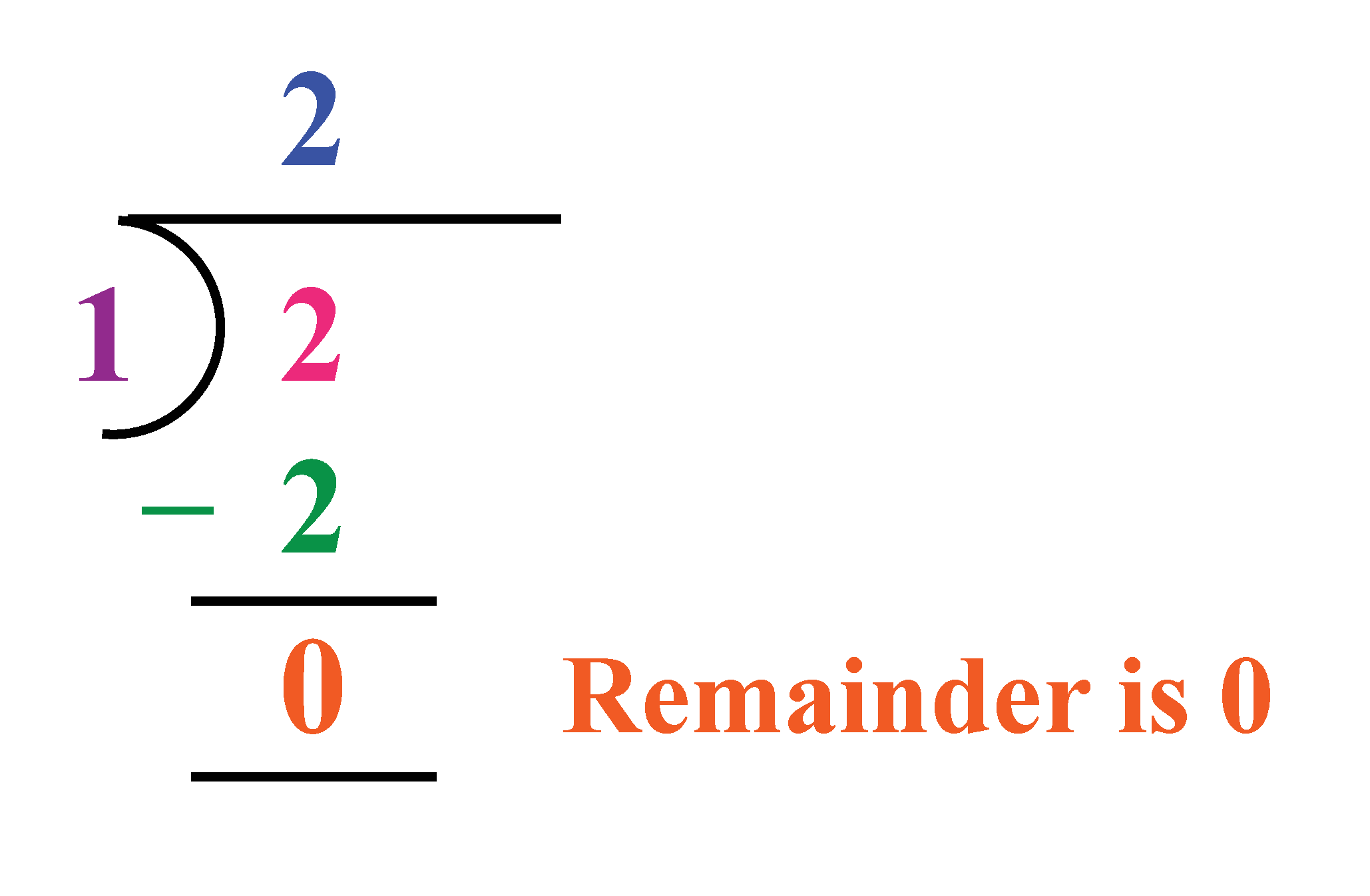While dividing a number, if the decimal expansion continues and the remainder does not become zero, it is called non-terminating.

Example:

\begin{align}\frac{1}{3} = 0.33333....\end{align} is a recurring, non-terminating decimal.

You can notice that the digits in the quotient keep repeating.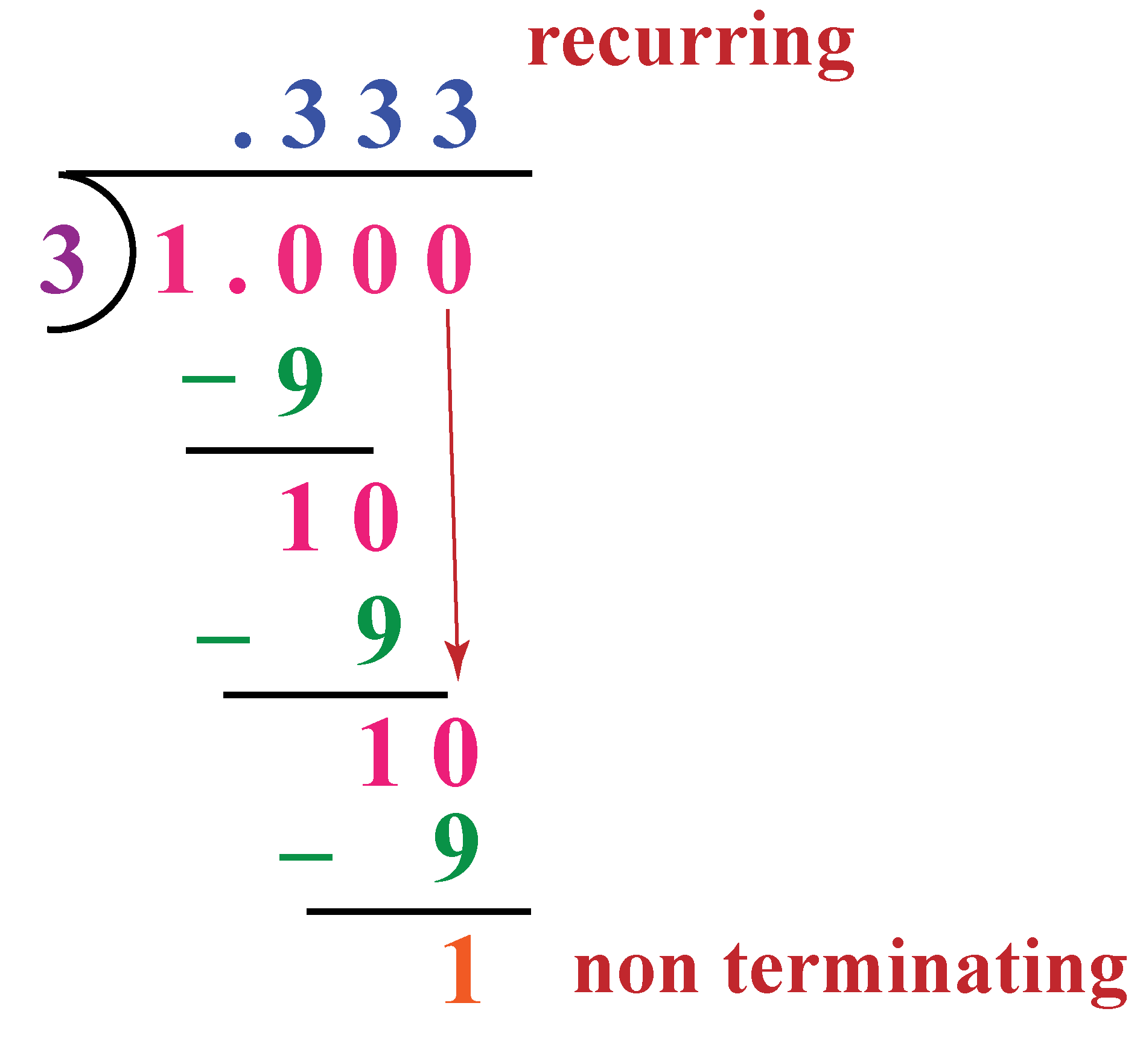If the decimal expansion is non-terminating and non-recurring, it is an irrational number.

## Non-Terminating Decimal and Terminating Decimal Representation

### Terminating Decimal Expansion

The terminating decimal expansion means that the decimal representation or expansion terminates after a certain number of digits.

For example: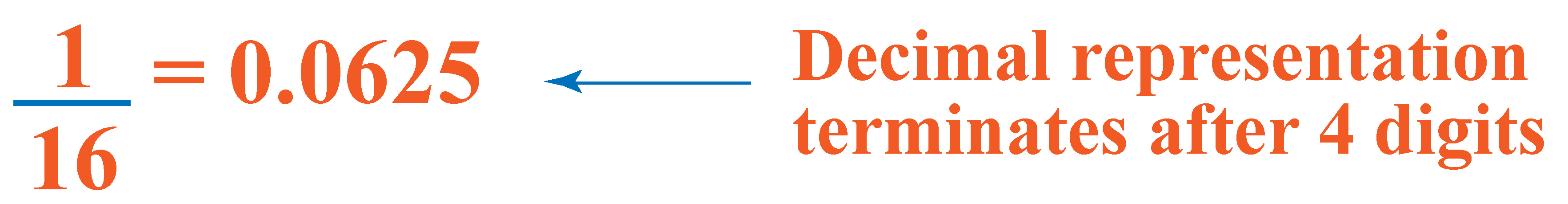Here, the decimal expansion of \begin{align}\frac{1}{{16}}\end{align} terminates after 4 digits.

Note that in terminating decimal expansion, you will find that the prime factorization of the denominator has no other factors other than 2 and 5.

### Non-terminating but Repeating Decimal Expansion

The non-terminating but repeating decimal expansion means that although the decimal representation has an infinite number of digits, there is a repetitive pattern to it.

For example: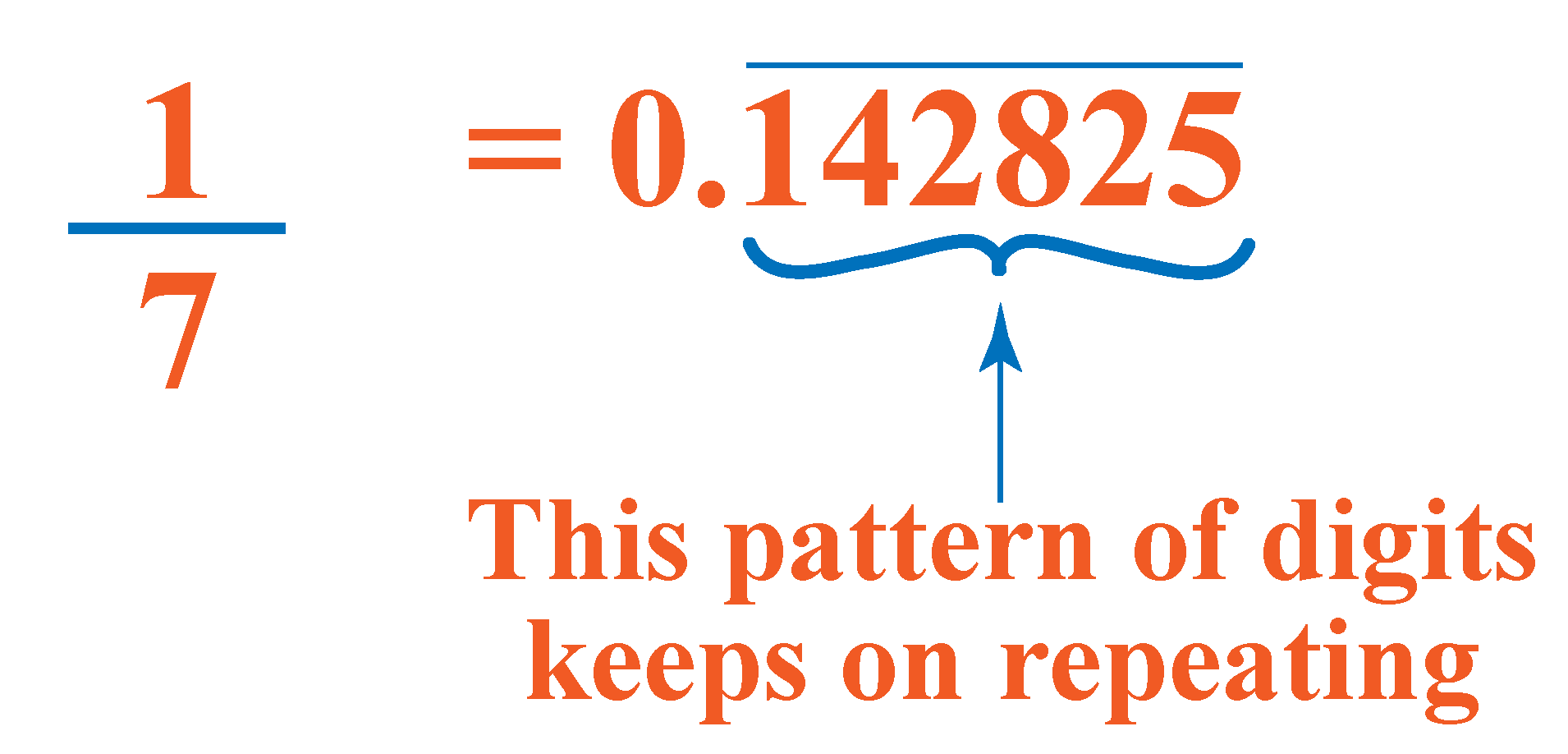In non-terminating but repeating decimal expansion, you will find that the prime factorization of denominator has factors other than 2 and 5.

Here is a small activity for you . In the simulation below check if the given rational number has a terminating or non -terminating decimal expansion.

## How Decimal Expansions Correspond to a Rational Number

It is easy to see why a terminating decimal representation corresponds to a rational number.

Consider a number $${x}$$ which has a terminating decimal representation with a certain number of digits (say $${n}$$) after the decimal point.

We take a particular example, with $${n}$$ equal to 5:

$x = 1.23867$

We can convert this into a rational form easily.

Remove the decimal point, and divide by 10 raised to the power $${n}$$ (or 1 followed by $${n}$$ zeroes):

$x = \frac{{123867}}{{{{10}^5}}} = \frac{{123867}}{{100000}}$

However, it is not so easy to see why a non-terminating but repeating decimal representation is also rational.

To understand this, check the Solved examples section.Tips and Tricks
1.  In terminating decimal expansion, the prime factorization of the denominator has no other factors other than 2 and 5
2.  In non-terminating but repeating decimal expansion, you will find that the prime factorization of the denominator has factors other than 2 and 5
3. Think: What type of numbers will represent non-terminating, non-repeating decimal expansions?

Help your child score higher with Cuemath’s proprietary FREE Diagnostic Test. Get access to detailed reports, customised learning plans and a FREE counselling session. Attempt the test now.

## Solved Examples

 Example 1

Express \begin{align}\frac{1}{13}\end{align} in decimal form.

Solution:

Let's divide 1 by 13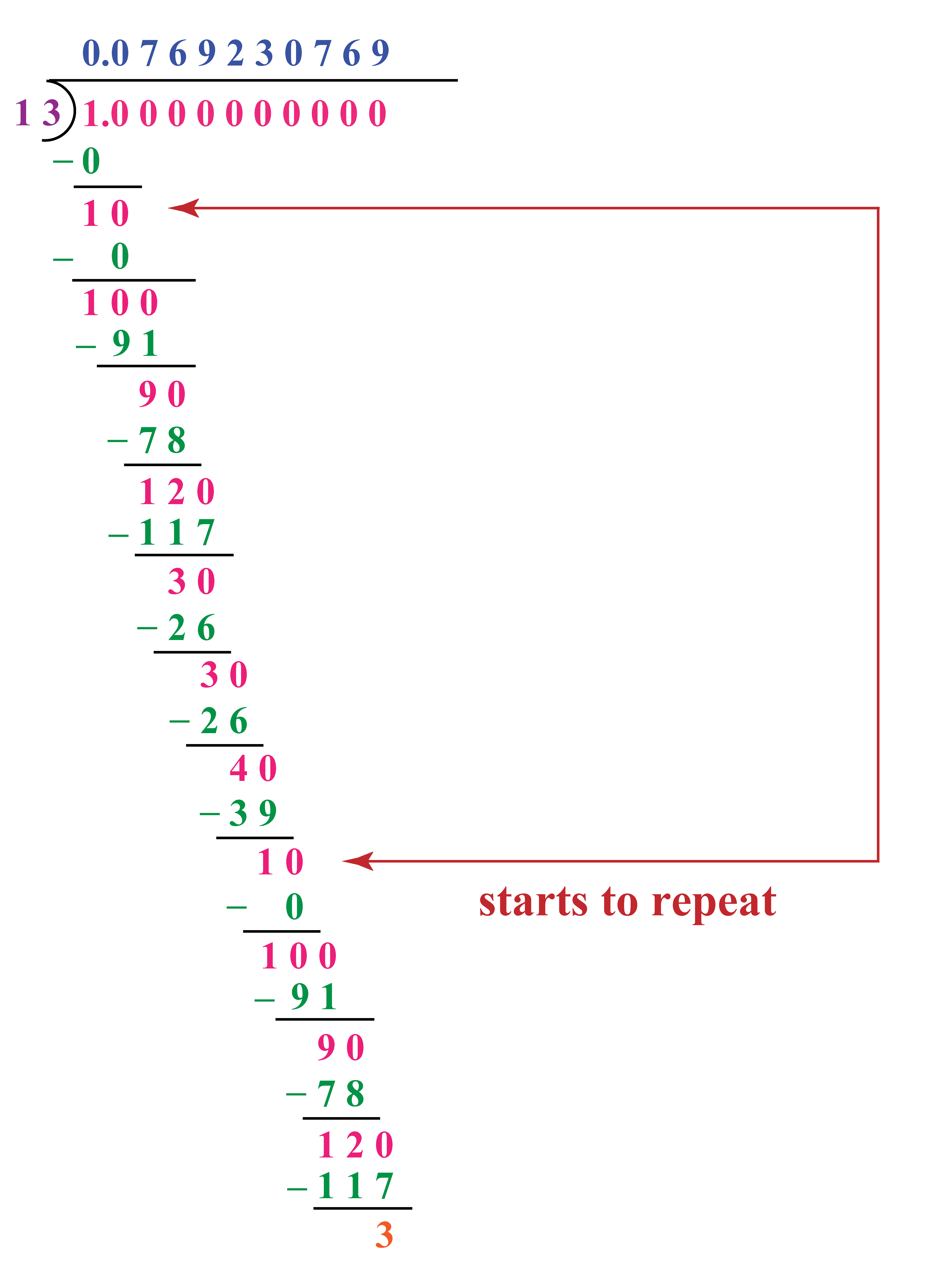We see that the quotient is 0.0769230769...which is a recurring decimal quotient.

 \begin{align}\therefore \frac{1}{13} =0.\overline{076923} \end{align}
 Example 2

Determine if \begin{align}\frac{11}{25}\end{align} is a terminating or a non-terminating number.

Solution:

A rational number is terminating if it can be expressed in the form \begin{align} \frac{p}{2^n \times 5^m}\end{align}

The prime factorisation of 25 is $$5 \times 5$$

\begin{align}\frac{11}{25} = \frac{11}{2^0 \times 5^2} \end{align}

Thus, \begin{align}\frac{11}{25}\end{align} is a terminating rational number.

 \begin{align}\therefore \frac{11}{25}\end{align} is a terminating number.
 Example 3

Express \begin{align}\frac{1}{27}\end{align}  using the recurring decimal form of  \begin{align}\frac{1}{3} =0.33\overline{3} \end{align}

Find the value of \begin{align}\dfrac{83}{27}\end{align}

Solution:

\begin{align}\frac{1}{27} = \frac{1}{9} \times \frac{1}{3}\end{align}

Given,

\begin{align}\frac{1}{3} =0.33\overline{3} \end{align}

\begin{align}\frac{1}{27} = \frac{1}{9} \times 0.33\overline{3} \end{align}

Dividing  $$\dfrac{0.333\overline{3}}{9}$$ we get, a recurring decimal $$0.\overline{037}$$

\begin{align} \frac{83}{27} = 3 \frac{2}{27}\end{align}

We can rewrite it as:

\begin{align}3 + \frac{2}{27} = 3 +2 \times \frac{1}{27}\end{align}

Simplifying further,

\begin{align} &=3+2 \left( \frac{1}{27} \right)\\\\ &=3 + 2 \times 0.\overline{037} \\\\ &=3 +0. \overline{074} \\\\ &=3.\overline{074} \end{align}

 $$\therefore \dfrac{83}{27} =3.\overline{074}$$
 Example 4

Convert the following into a rational form:

$x = 0.343434...$

Solution:

We have,

\begin{align} x &= 0.343434 \ldots \\ \Rightarrow 100x &= 34.343434 \ldots \end{align}

Subtracting these two, we have,

$99x = 34$

Or,

\begin{align}x = \frac{34}{99}\end{align}

 \begin{align}\therefore x = \frac{34}{99} \end{align}
 Example 5

Convert the following into a rational form:

$y = 1.721873873873...$

Solution:

We have,

$y = 1.721873873873...$

Multiplying by 1000 and 1000000, we get,

$\Rightarrow \left\{ {\begin{array}{*{20}{l}} {1000y = 1721.873873873...} \\ {1000000y = 1721873.873873873...} \end{array}} \right.$

Subtracting these two, we have,

\begin{align} \left( {1000000 - 1000} \right)y &= 1721873 - 1721 \hfill \\ \Rightarrow 999000y &= 1720152 \hfill \\ \end{align}

$\Rightarrow y = \frac{1720152}{999000}$

 \begin{align}\therefore y = \frac{{1720152}}{{999000}}\end{align}Challenging Questions
1.  Without performing long division, state whether the following rational numbers will have a terminating decimal expansion or a non-terminating but repeating decimal expansion

$\frac{{129}}{{{2^2}{5^7}{7^5}}},\frac{6}{{15}},\frac{{77}}{{210}}$

2. Convert $$0.9999...$$ into a rational number. Are you surprised? (Tip: Let $$x = 0.9999...$$ and then multiply $$10$$ on both sides)

Have a doubt that you want to clear? Get it clarified with simple solutions on Decimal Representation of Rational Numbers from our Math Experts at Cuemath’s LIVE, Personalised and Interactive Online Classes.

Make your kid a Math Expert, Book a FREE trial class today!

## Practice Questions

Here are a few activities for you to practice.

IMO (International Maths Olympiad) is a competitive exam in Mathematics conducted annually for school students. It encourages children to develop their math solving skills from a competition perspective.

## 1. Is a decimal a rational number?

Terminating and repeating decimal numbers are rational numbers.

## 2. How do you know if a decimal is rational?

If a decimal number can be expresed in the form  $$\frac{p}{q}$$  and $$q \neq 0$$, it is a rational number.

Example: $$0.25 = \dfrac{25}{100}$$ is a rational number.

## 3. What are the characteristics of a rational number when written as a decimal?

When expressing a rational number in the decimal form, it can be terminating or non terminating and the digits can recur in a pattern.

Example: \begin{align}\frac{1}{2} = 0.5 \end{align} is a terminating decimal number.

\begin{align}\frac{1}{3} = 0.33333...\end{align} is a non-terminating decimal number with the digit 3 repeating.

If it is non-terminating and non-recurring, it is not a rational number.

Example: $$\pi$$

## 4. Is every decimal number represented as a rational number?

Non-terminating and non-repeating digits to the right of the decimal point cannot be expressed in the form $$\frac{p}{q}$$ hence they are not rational numbers.

## 5. Is 3.14 a rational number?

A rational number is a number that can be written as a fraction, $$\frac{a}{b}$$ where a and b are integers.

Hence, the number 3.14 is a rational number.

## 6. How to know if a number is irrational?

Irrational number cannot be expressed in the form $$\frac{p}{q}$$

It has endless non-repeating digits after the decimal point.

Examples:  $$\pi = 3.141592…$$ , $$\sqrt{2}= 1.414213…$$

## 7. How do you convert a rational number to a decimal?

To convert fractions to decimals, just divide the numerator by the denominator.

If the division doesn't end evenly, we can stop after a certain number of decimal places and round it off.

## 8. Is a decimal an integer?

No, they are both different.

Decimals refer to a number system in base of 10, which means it is written using the digits between 0 to 9.

Integers on the other hand are a set of numbers that include natural numbers, their negatives and 0.

Numbers and Number Systems
Numbers and Number Systems
grade 9 | Questions Set 2
Numbers and Number Systems